# GMAT Math : Sectors

## Example Questions

← Previous 1 3 4

### Example Question #31 : Circles

Brendan's girlfriend made him a cheescake for his birthday. He eats one slice a day. What is the measure of the central angle of each slice?

I) The diameter of the cake is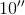.

II) Each slice is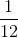of the total cake.

Statement II is sufficient to answer the question, but Statement I is not sufficient to answer the question.

Either statement alone is sufficient to answer the question.

Statement I is sufficient to answer the question, but Statement II is not sufficient to answer the question.

Both statements together are needed to answer the question.

Statement II is sufficient to answer the question, but Statement I is not sufficient to answer the question.

Explanation:

In this case we are given a circle and asked to find the angle of a portion of it.

The diameter would allow us to find many things related to the circle, but not an individual slice.

However, knowing that each slice is 1/12 of the total allows us to multiply 360 by 1/12 and find out that each slice is 30 degrees.

Therefore statement II alone is sufficient in answering the question.

### Example Question #31 : Circles

One slice of a pizza is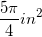. What is the central angle of one slice?

I) Each slice isof the whole pizza.

II) Each straight edge the slice is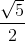inches.

Either statement alone is sufficient to answer the question.

Statement II is sufficient to answer the question, but Statement I is not sufficient to answer the question.

Both statements together are needed to answer the question.

Statement I is sufficient to answer the question, but Statement II is not sufficient to answer the question.

Either statement alone is sufficient to answer the question.

Explanation:

I) Gives us the percentage of one slice of the whole pizza. We can take 15% of 360 to find the central angle.

II) Gives us the radius of the pizza. We can use the radius to find the area of the pizza. With the total area and the area of one slice we can find the percentage of the whole and from there, the angle of one slice.

### Example Question #472 : Geometry

Walder is at his cousin's wedding preparing to eat a slice of pie.

I) Walder's slice has a radius of half a meter

II) Walder's slice is 5% of the total pie

What is the central angle of Walder's slice?

Statement I is sufficient to answer the question, but Statement II is not sufficient to answer the question.

Either statement is sufficient to answer the question.

Statement II is sufficient to answer the question, but Statement I is not sufficient to answer the question.

Both statements are needed to answer the question.

Statement II is sufficient to answer the question, but Statement I is not sufficient to answer the question.

Explanation:

The central angle of a sector, in this case represented by the slice of pie, can be thought of as a percentage of the whole circle. Circles have 360 degrees.

Statement I gives us the radius of the circle. We could find the diameter, area, or circumference with this, but not the central angle of that slice.

Statement II gives us the percentage of the whole circle that the slice represents, 5%. We can use this to find the number of degrees in the central angle of the slice, because it will just be 5% of 360.

Thus, Statement II is sufficient, but Statement I is not.

To recap:

Walder is at his cousin's wedding preparing to eat a slice of pie.

I) Walder's slice has a radius of half a meter

II) Walder's slice is 5% of the total pie

What is the central angle of Walder's slice?

Use Statement II to find the angle. The angle must be 5% of 360: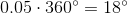### Example Question #1 : Dsq: Calculating The Angle For A Percentage Of A Circle

Alex decided to order a circular pizza. Find the angle that represents the slice of pizza he ate.

II) The slice Alex ate represented one-fifth of the total pizza.

Both statements are needed to answer the question.

Either statement is sufficient to answer the question.

Statement I is sufficient to answer the question, but statement II is not sufficient to answer the question.

Statement II is sufficient to answer the question, but statement I is not sufficient to answer the question.

Statement II is sufficient to answer the question, but statement I is not sufficient to answer the question.

Explanation:

To find the angle of a sector (in this case, that represented by the slice of pizza), we need to know with how much of the circle we are dealing.

Statement I gives us the radius of the circle. This is helpful for a lot of other things, but not finding our central angle.

Statement II tells us what portion of the pizza we are concerned with. We can multiply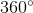by one-fifth to get the correct answer.

Using Statement II if the slice is one-fifth of the total pizza, then we can do the following to find the answer: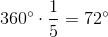Thus, Statement II is sufficient, but Statement I is not.

### Example Question #1 : Sectors

Find the angle for the percentage of a circle.

Statement 1:  A circle diameter is 5.

Statement 2:  The sector length is.

Statement 1 ALONE is sufficient, but Statement 2 ALONE is not sufficient to answer the question.

BOTH statements TOGETHER are NOT sufficient, and additional data is needed to answer the question.

Statement 2 ALONE is sufficient, but Statement 1 ALONE is not sufficient to answer the question.

BOTH statements taken TOGETHER are sufficient to answer the question, but neither statement ALONE is sufficient.

EACH statement ALONE is sufficient.

Statement 1 ALONE is sufficient, but Statement 2 ALONE is not sufficient to answer the question.

Explanation:

The question asks to solve the angle of a percentage of a circle.

Statement 1):  A circle diameter is 5.

Statement 1) is sufficient to solve for the angle of the circle because the statement itself provides that the shape is a full circle, 360 degrees, and is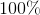of the circular sector.

Statement 2):  The sector length is.

Statement 2) does not have sufficient information to solve for the angle.  The precentage of the circle is not provided and we do not know how much of the circle will have a sector length of.  We also cannot assume that the sector is a full circle to make any further conclusions.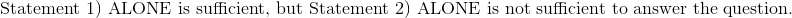### Example Question #1 : Sectors

Dirk bought a sticker to cover part of the base of his scuba tank. The sticker and the base have the same radius. Given the following, find the percentage of the base that the sticker covers.

I) The central angle of the sticker is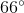II) The base of the tank has a radius of 5 inches.

Statement II is sufficient to answer the question, but Statement I is not sufficient to answer the question.

Statement I is sufficient to answer the question, but Statement II is not sufficient to answer the question.

Both statements are needed to answer the question.

Either statement is sufficient to answer the question.

Statement I is sufficient to answer the question, but Statement II is not sufficient to answer the question.

Explanation:

To find the percentage that the sticker covers, we need to know what portion of the circle it covers. We could find this either with the area of the circle or the area of the sector, but we don't have a good way to find the area of the sector.

Statement I gives us the degree measurement of the sticker. We can make a fraction,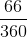, to find the percentage of the base covered.

Statement II gives us the radius of the base, which lets us also know the radius of the sticker; however, this will not be helpful in finding the percentage of the base covered, so Statement II is not really helpful.

Thus, Statement I is sufficient and Statement II is not.

Recap:

Dirk bought a sticker to cover part of the base of his scuba tank. The sticker and the base have the same radius. Given the following, find the percentage of the base that the sticker covers.

I) The central angle of the sticker isII) The base of the tank has a radius of 5 inches

So, according to Statement I, the angle of the sticker is 66 degrees. Because we are dealing with a circle, we can find the percentage of the circle covered by putting 66 over 360.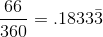The sticker covers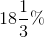of the scuba tank.

### Example Question #1 : Sectors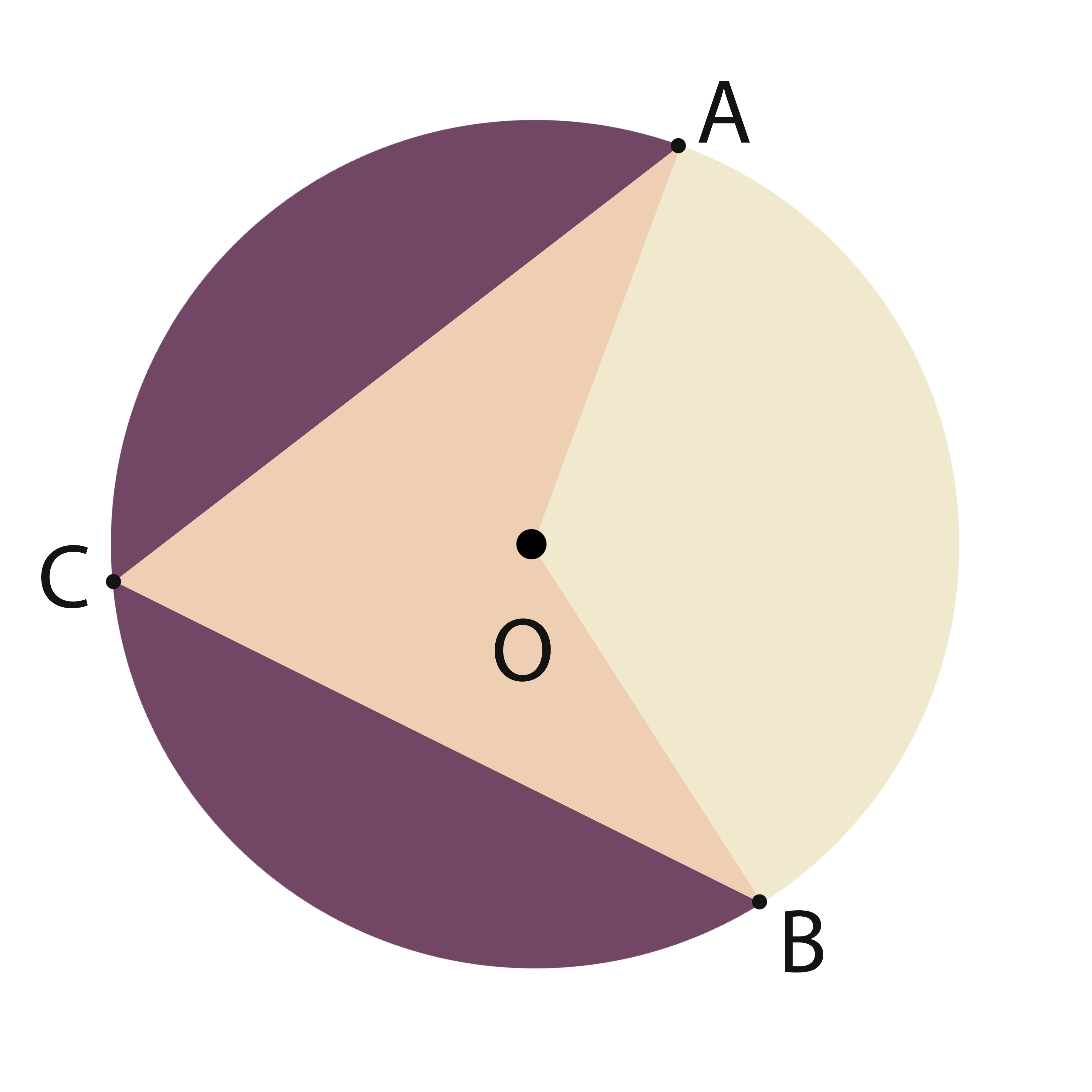What is the area of sector AOB?

1)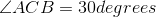2) Arclength of AB is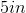Neither of the statements, separate or together, is sufficient.

Statement 1 alone is sufficient.

Together the two statements are sufficient.

Statement 2 alone is sufficient.

Either of the statements is sufficient.

Together the two statements are sufficient.

Explanation:

The angle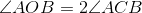, so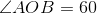.

The Arc length can be related to the radius as follows: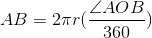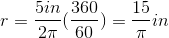From there, the area of the sector can be found: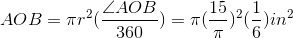### Example Question #41 : Circles

Consider circle F with sector BFG. What percent of F is BFG?

I) Circle F has a radius of 5 meters and a circumference of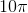meters.

II) Sector BFG has a central angle measure of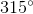.

Statement I is sufficient to answer the question, but statement II is not sufficient to answer the question.

Both statements are needed to answer the question.

Statement II is sufficient to answer the question, but statement I is not sufficient to answer the question.

Either statement is sufficient to answer the question.

Statement II is sufficient to answer the question, but statement I is not sufficient to answer the question.

Explanation:

Consider circle F with sector BFG. What percent of F is BFG?

I) Circle F has a radius of 5 meters and a circumference ofmeters

II) Sector BFG has a central angle measure ofTo find the percentage of a circle covered by a sector, we need to know the central angle. A circle will always have, so if we put the angle given to us over. So, II) allows us to answer the question: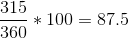So sector BFG is 87.5% of circle F

I) Give us interesting information, but not helpful in regards to the current question. It doesn't matter what the radius is, and the circumference is also irrelevant.

Therefore, II) is sufficient, but I) is not.

### Example Question #1 : Dsq: Calculating The Percentage Of A Sector From An Angle

Jane wants to put a sector-shaped sticker on the bottom of her cylindrical water bottle. The water bottle has a circular base. Find the percent of the base that the sticker covers

I) The sticker has a thickness of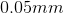.

II) The central angle of the sticker has a measure of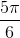.

Statement II is sufficient to answer the question, but statement I is not sufficient to answer the question.

Statement I is sufficient to answer the question, but statement II is not sufficient to answer the question.

Either statement is sufficient to answer the question.

Both statements are needed to answer the question.

Statement II is sufficient to answer the question, but statement I is not sufficient to answer the question.

Explanation:

Jane wants to put a sector-shaped sticker on the bottom of her cylindrical water bottle. The water bottle has a circular base. Find the percent of the base that the sticker covers

I) The sticker has a thickness ofII) The central angle of the sticker has a measure ofI) is irrelevant. The thickness of  the sticker doesn't help us find the area it covers. Statment I) is trying to distract you with 3 dimensions, when we only need to worry about 2.

II) is much more helpful. If we know the central angle, we can find the percentage the sector covers. To find the "answer" perform the following: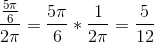So we are dealing with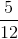of the circle, or 41.67%

### Example Question #1 : Dsq: Calculating The Area Of A Sector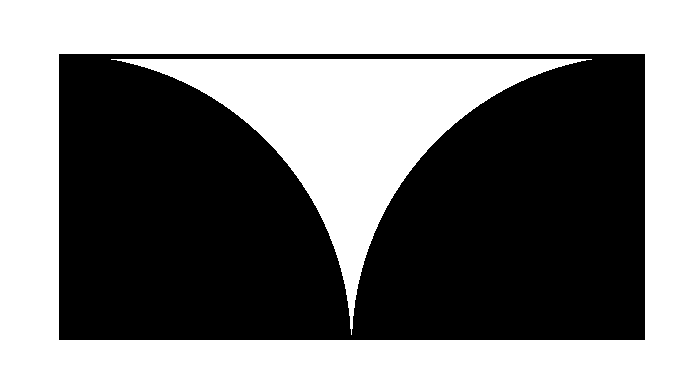The above figure shows two quarter circles inscribed inside a rectangle. What is the total area of the white region?

Statement 1: The area of the black region is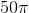square centimeters.

Statement 2: The rectangle has perimeter 60 centimeters.

Statement 1 ALONE is sufficient to answer the question, but Statement 2 ALONE is NOT sufficient to answer the question.

EITHER statement ALONE is sufficient to answer the question.

BOTH statements TOGETHER are insufficient to answer the question.

BOTH statements TOGETHER are sufficient to answer the question, but NEITHER statement ALONE is sufficient to answer the question.

Statement 2 ALONE is sufficient to answer the question, but Statement 1 ALONE is NOT sufficient to answer the question.

EITHER statement ALONE is sufficient to answer the question.

Explanation:

The width of the rectangle is equal to the radius of the quarter circles, which we call; the length is twice that, or.

The area of the rectangle is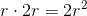; the total area of the two black quarter circles is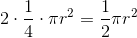, so the area of the white region is their difference,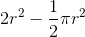Therefore, all that is needed to find the area of the white region is the radius of the quarter circle.

If we know that the area of the black region iscentimeters, then we can deduceusing this equation: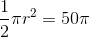If we know that the perimeter of the rectangle is 60 centimeters, we can deducevia the perimeter formula: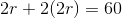Either statement alone allows us to find the radius and, consequently, the area of the white region.

← Previous 1 3 4

### All GMAT Math Resources